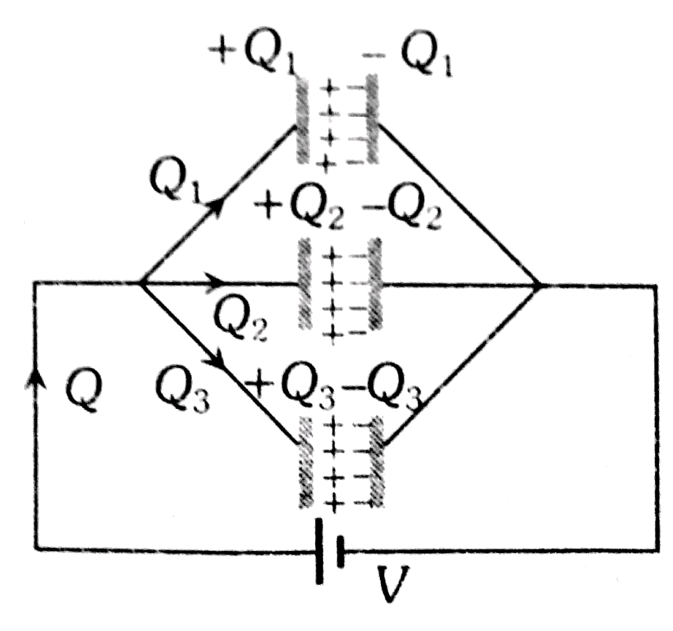Q

# Please help! Two capacitors C1 and C2 are charged to 120 V and 200 V respectively. It is found that by connecting them together the potential of each one can be made zero. Then :

Two capacitors C1 and C2 are charged to 120 V and 200 V respectively. It is found that by connecting them together the potential of each one can be made zero. Then :

• Option 1)

9C1 = 4C2

• Option 2)

5C1 = 3C2

• Option 3)

3C1 = 5C2

• Option 4)

3C1 + 5C2 = 0

147 Views
N

As we discussed in

Parallel Grouping -

- whereinOption 1)

9C1 = 4C2

Option 2)

5C1 = 3C2

Option 3)

3C1 = 5C2

Option 4)

3C1 + 5C2 = 0

Exams
Articles
Questions